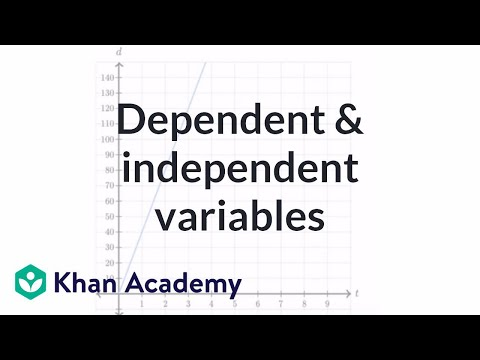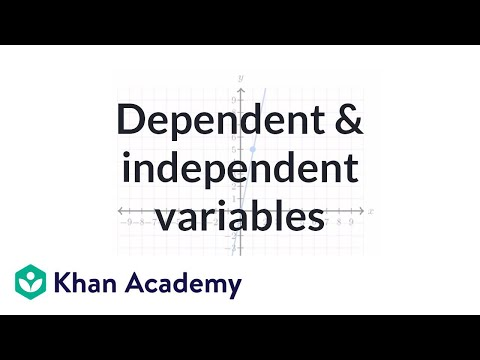Video

# Dependent & independent variables (Full video)

Description: Identifying the dependent and the independent variables in a word problem. Created by Sal Khan. In the table above, q represents the number of questions that you answer correctly on your math quiz, and p represents the total number of points that you score on your quiz. The relationship between these two variables can be expressed by the following equation. p is equal to 5q, where p is the points you get and q is the number of questions you answered correctly. So when you think about what's happening here, is your number of points you score is being driven by how many questions you get right.

### Other videos you might be interested in### Dependent & independent variables: equation (Full video)